# Boole

Also found in: Dictionary, Thesaurus, Wikipedia.

## Boole

George. 1815--64, English mathematician. In Mathematical Analysis of Logic (1847) and An Investigation of the Laws of Thought (1854), he applied mathematical formulae to logic, creating Boolean algebra
Collins Discovery Encyclopedia, 1st edition © HarperCollins Publishers 2005

## Boolean logic

The "mathematics of logic," developed by English mathematician George Boole in the mid-19th century. Just as add, subtract, multiply and divide are the primary operations of arithmetic, AND, OR and NOT are the primary logical operators of Boolean logic and building blocks of every digital circuit. NAND (not AND), NOR (not OR) and XOR (exclusive OR) are variations of AND, OR and NOT (see NAND, NOR and XOR). See Boolean search, binary, logic gate and Bebop to the Boolean Boogie.

Decimal vs. Binary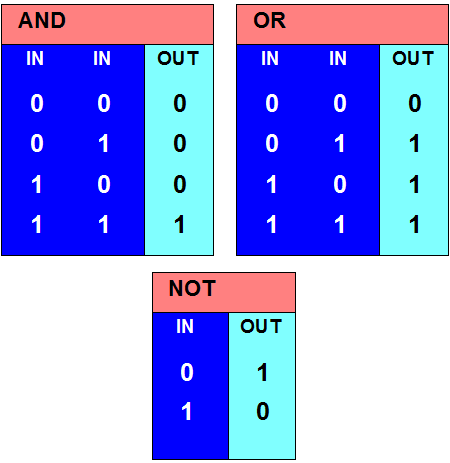Decimal arithmetic holds 10 values (0 to 9) in each digit position. In binary, there are only two (0 and 1). Note below the four possible result and carry bits when adding two binary digits (bits) together.

AND, OR and NOT Gates
Transistors wired in series and parallel patterns make up these "gates," which accept inputs of 0 (no pulse) or 1 (a pulse) and generate outputs of 0 or 1. While AND requires that both inputs are 1 to generate an output of 1, OR only needs one input to be 1. NOT reverses the input. For a diagram of these actions, see Boolean gates.

Decimal vs. Binary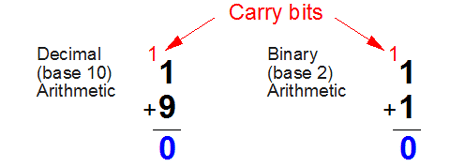Decimal arithmetic holds 10 values (0 to 9) in each digit position. In binary, there are only two (0 and 1). Note below the four possible result and carry bits when adding two binary digits (bits) together.

Add a One and Zero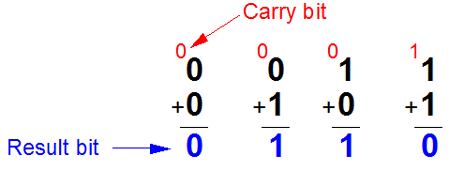Trace the red 1 (pulse, current) and white 0 (no pulse, no current) through the gates and notice their outputs. This half-adder circuit is in every CPU.

Add a One and Zero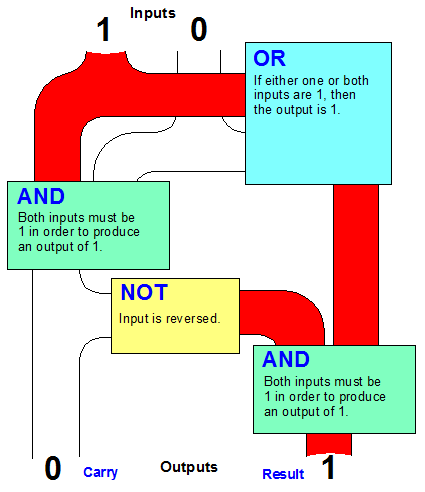Trace the red 1 (pulse, current) and white 0 (no pulse, no current) through the gates and notice their outputs. This half-adder circuit is in every CPU.

Trace the Flow YourselfChoose any two binary digits as input and trace them through this half-adder circuit (0 is no current; 1 is current). Watch how the output is generated.

Trace the Flow Yourself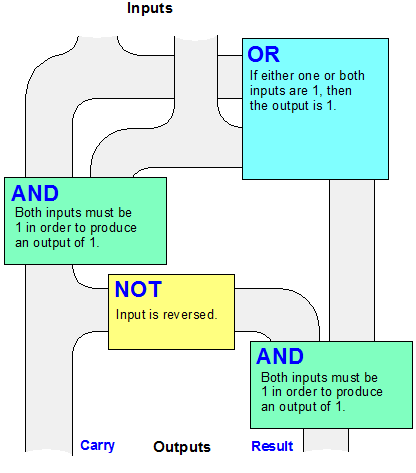Choose any two binary digits as input and trace them through this half-adder circuit (0 is no current; 1 is current). Watch how the output is generated.

Patterns of Boolean Logic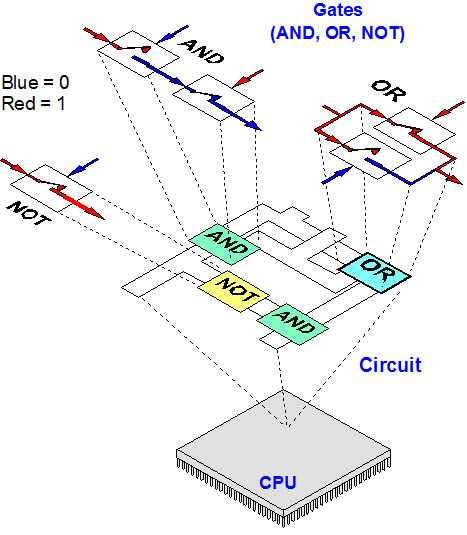Transistors (depicted here as mechanical switches) make up gates. Gates make up circuits, and circuits make up every digital device. There are millions and billions of transistors in modern chips, and they fit in an area smaller than a postage stamp. For more details, see Boolean gates. See transistor and chip.
Copyright © 1981-2019 by The Computer Language Company Inc. All Rights reserved. THIS DEFINITION IS FOR PERSONAL USE ONLY. All other reproduction is strictly prohibited without permission from the publisher.
References in periodicals archive ?
A computer is, at its heart, simply an instantiation in electronic circuitry of the logical algebra worked out by Boole. Since logic is the systematization of deductive reasoning, which is an activity of the brain, the question irresistibly arises: how similar to what the human brain does is what the computer does?
The financial analysts at Merrill Lynch suggest BMC's 25% core growth targets for the current fiscal year will be exceeded "on a combination of new product flow and realized synergies with Boole and New Dimension."